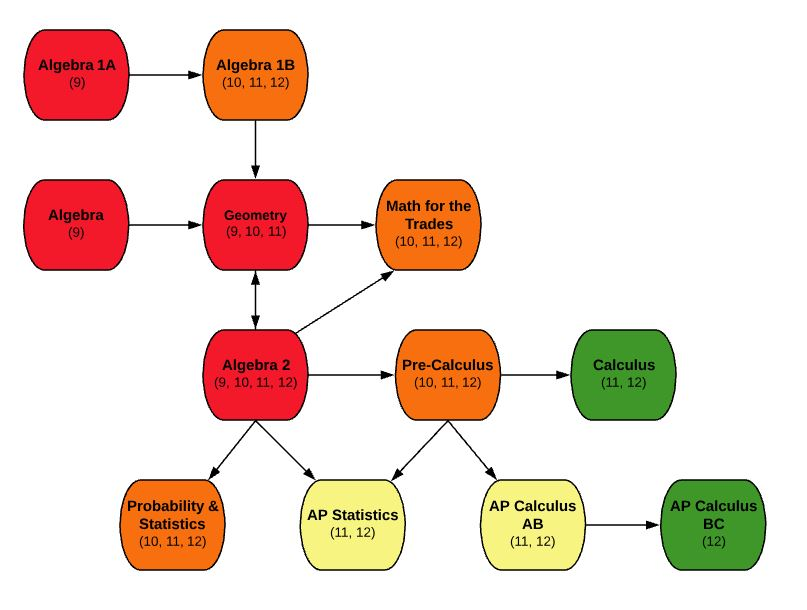EDIT MAIN

# MA310 Probability & Statistics10, 11, 12

Mathematics

Standard

1

This is a year long course that requires selection of both semesters: S1 and S2. This is a project based course designed to introduce students to the four themes of statistics, exploring data, sampling and experimentation, anticipating patterns and statistical inference. Real world applications will be a primary emphasis. Students will also be incorporating the use of technology and problem solving skills.

Outcomes:

• Construct and interpret distributions with graphs, charts, and tables
• Describe and compare distributions
• Calculate numerical summaries by hand and using technology
• Describe and interpret locations in a distribution (z-scores, percentiles, etc.)
• Perform Normal distribution calculations including assessing normality
• Construct and interpret scatterplots
• Measure, calculate, and interpret correlation and a least square regression line -Interpret computer output
• Describe different sampling methods (stratified, cluster, etc.)
• Conduct simple random samples (using a random number table and technology)
• Design comparative experiments (completely randomized, randomized block, and matched pairs designs)
• Use rules of probability to construct venn diagrams, two-way tables, and determine probabilities
• Apply the concept of discrete random variables to many different statistical settings
• Calculate the expected values of a discrete random variable
• Describe continuous random variables
• Compute probabilities involving binomial and geometric random variables

MA300-S1 – Algebra II S1 AND MA300-S2 – Algebra II S2

If you take this course, you must also take MA310-S2 – Probability & Statistics S2